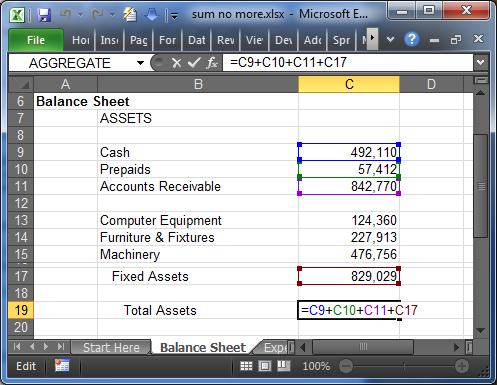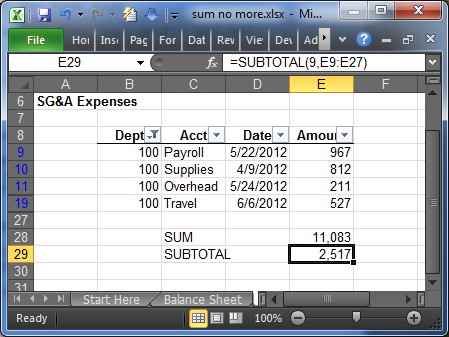# SUM() No More

What is your #1 most-used Excel worksheet function? Is it the SUM function? Believe it or not, there is an Excel function that is superior to SUM and once you discover it, you’ll hardly use SUM again.

I’m referring to the SUBTOTAL worksheet function and if you haven’t explored it yet, I would strongly encourage you to play with it and use it as a replacement for SUM. This function has the ability to dramatically improve both the accuracy and efficiency of your workbooks. Ready to see it in action? Let’s get started.

## SUBTOTAL

I think using the word “always” is a bad idea, so I’ll say this: I almost always use the SUBTOTAL function instead of the SUM function.

Why? The key to the SUBTOTAL function is that it excludes other SUBTOTAL functions in its range. I’ll restate this key point, but this time, with feeling. The SUBTOTAL function excludes other SUBTOTAL functions from its result. If the beauty of that hasn’t settled in yet, let me illustrate.

Let’s consider a typical balance sheet. A fairly standard approach to computing a subtotal, such as the Fixed Assets subtotal, is by using the SUM function, as shown in cell C17 in Figure 1 below.Figure 1

Now that the Fixed Assets subtotal is computed, we’ll move down to the Total Assets computation. A fairly standard approach is to write a formula that references each individual cell to be included in the total, as shown in cell C19 in Figure 2 below.Figure 2

And that, my friend, is a fairly common formula approach to preparing balance sheets and other Excel reports.

So, what’s wrong with that? The approach is inefficient and prone to errors.

It is error-prone, because as soon as a new item is inserted into the balance sheet, such as Inventory, the balance sheet no longer foots. And, thus, the Total Assets formula must be updated or rewritten, which is inefficient.

Let’s see why using SUBTOTAL instead of SUM is a better approach. For the Fixed Assets subtotal, rather than use the SUM function, as we did in Figure 1, we’ll use a SUBTOTAL function, as shown in cell C17 in Figure 3 below.Figure 3

Now, instead of picking and choosing which cells are included in Total Assets as we did previously, we can simply use a SUBTOTAL function to add up the entire range of assets, as shown in cell C19 in Figure 4 below.Figure 4

If you are concerned that Fixed Assets will be double-counted, don’t be. Remember, the key feature of the SUBTOTAL function is that it excludes all other SUBTOTAL functions in its range. Thus, it will not double-count Fixed Assets since the Fixed Assets total was computed with a SUBTOTAL function.

In our example above, the SUBTOTAL function is used for Total Assets and all other subtotals such as Fixed Assets. As we insert new items, we know that (1) the report will continue to foot and (2) we won’t have to update the Total Assets formula. As you can probably tell, SUBTOTAL is a much better approach than SUM functions in many situations.

## Arguments

Do you know what “function arguments” are? Function arguments are those values or cell references placed between the parentheses after the function name. They are used by the function to compute the results.

For example, the SUM function accepts a function argument that represents the range to sum.

The SUBTOTAL function has two function arguments. The first argument is a code (e.g., 9, 1,2) that tells the SUBTOTAL function what kind of math to use (e.g., sum, average, count). The second argument is the range. 9 is the only code I use and have memorized, because I only use the SUBTOTAL function for adding ranges. So, all of my SUBTOTAL functions are similar to the one used in Figure 4 above, which follows:

=SUBTOTAL(9,C9:C18)

Where:

• 9 is the function_num code for add (sum)
• C9:C18 is the range to add

If instead of adding the range you want to find the average of the range, you would use 1 for the first argument, for example, SUBTOTAL(1, range). For a list of all function numbers, please refer to the Excel help system.

## Better Together

In addition to making your reports more bulletproof, try using the SUBTOTAL function with filtered data. When you enable Filters on a range, you’ve probably noticed that the SUM function includes all rows in the filtered range, whether or not those rows are visible. The SUBTOTAL function however, only includes rows that are visible in filtered data tables, as demonstrated in cell E29 in Figure 5 below.Figure 5

Thus, using the SUBTOTAL function in conjunction with filtered data allows you to perform easy analysis and create interactive reports.

I hope you’ll find as much use out of the SUBTOTAL function as I have over the years, and I hope that it enables you to do your work more efficiently and more accurately.

Now, go and SUM no more.

Posted in### Jeff Lenning

I love sharing the things I've learned about Excel, and I built Excel University to help me do that. My motto is: Learn Excel. Work Faster.

### Excel is not what it used to be.

You need the Excel Proficiency Roadmap now. Includes 6 steps for a successful journey, 3 things to avoid, and weekly Excel tips.